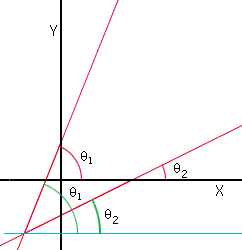Subject: Precalculus: Angle of Intersection of Two Lines Name: Veronica Patterson Who is asking: Student Level: Secondary Question: I am having a real hard time trying to figure out this problem. Could you please help me! The homework question says to find the acute angle of intersection between the two lines y=3x+1 and y=(1/2)x-1. (It also says to use the results of a problem I had already figured out.) That problem was to use information from a picture shown that tan(theta sub1-theta sub2)= ((m sub2- m sub1)/(1+(m sub1 * m sub2))). I used the difference identity of tangent to figure out the answer. Any help on this problem would be greatly appreciated. Thanks. Veronica Patterson Hi again Veronica Since you say "I used the difference identity of tangent to figure out the answer." I assume you know that the slope of a line is the tangent of the angle, measured counterclockwise, between the X-axis and the line. I drew a diagram of the two lines and marked in red the angles theta1 anf theta2. The tangents of theta1 and theta2 give the slopes of the two lines.I then drew a horizontal line (in blue) through the intersection of the two lines and marked the angles again, this time in green. It should be clear now that the angle between the two lines is theta1-theta2. The slopes of the two lines are 3 and 1/2 so you can use the result from the previous problem to calculate tan(theta1-theta2). Once you know the value of tan(theta1-theta2) you should be able to deduce theta1-theta2. Cheers, Harley Go to Math Central